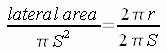SEARCH HOMEMath Central Quandaries & QueriesQuestion from ashley, a parent: What is the radius of a cone with the lateral area being 443.3 mm^2 and the slant height being 14.7 mm. Please help. the teacher gave her the following formula to use for the above S=pr^2+pra p=pi r=radius a=area Thank youHi Ashley,

The expression that the teacher gave is for the entire surface area of the cone. πr2 gives the area of the circular base and πra gives the area of the lateral region, the sloped sides. In this expression however the letter a designates the slant height. There is a development of this expression in Stephen's response to a question a while ago. Stephen uses slightly different notation and getswhere S is the slant height. Simplification of this expression yields

lateral area = π r S.

For your problem you know the lateral area and the slant height so

443.3 mm2 = 14.7 π r mm2

Solve for r.

PennyMath Central is supported by the University of Regina and The Pacific Institute for the Mathematical Sciences.